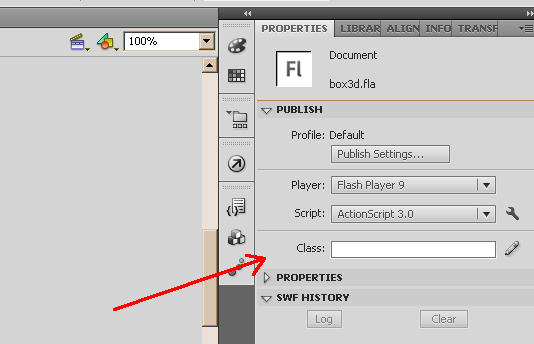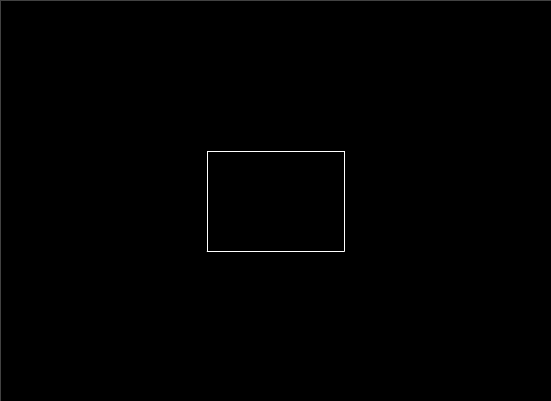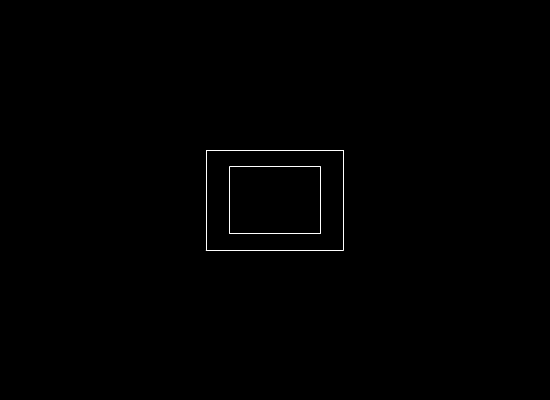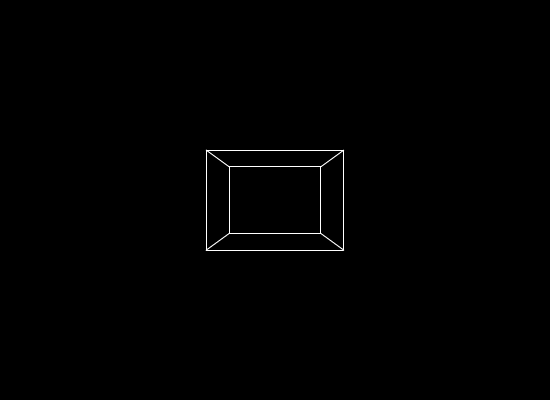This website uses cookies to improve user experience. By using our website you consent to all cookies in accordance with our Cookie Policy. X

### Basics of tile-based engine part 2: path finding [advanced]

In the previous part I've talking a little about isometric view and how to achieve it a simplest way possible. This time around, just as promised, we will have a look at path finding in tile-based engine using one of the easiest methods available (but not the fastest). I am not really sure if the algorithm presented here has any specific name, but without a doubt it is the most direct way out of all possible solutions.
For all of you that are not interested in details I've prepared a class built around the whole algorithm, plus also a FLA file showing how to work with it: PathFind.zip
Running it will look like this:
(Pick a starting and an ending point but clicking any empty field. SPACE to create different map)

The idea behind the algorithm is quite simple and can be divided into two phases:
1. Target search. Starting for on of the chosen points we check, step by step, every tile in the neighborhood in search of the second specified point, while constantly saving current iteration step into the grid. These numbers are needed in the next phase.
Here is a code snippet from the example:

```// ...
var vWrite:Vector.<Vector.<int>> = recreateTiles(tiles);
vWrite[startx][starty] = 1;
vWrite[endx][endy] = -1;
var vCheck:Vector.<Point>;

var tile:Point;
var nStep:int = 2;

for each(tile in vCheck) if(lookupTile(tile.x, tile.y, nStep, vRead,vWrite,vTile)) {
return retracePath(tile.x,tile.y,nStep,vWrite);
}
nStep ++;
}
return null;
// ...
```
The lookupTile function looks for a next tile to be processed. If the end destination is anywhere in the 4 directions from the current tile, the loop ends and a found path is returned. In other cases vWrite is updated to hold new information, while any found tile is written into vRead to be processed in next iteration.
2. Path backtracking. Once we find our destination it is time to backtrack along the numbers saved in vWrite ,one by one to retrieve our path. Lets say the iteration stopped at 20 step - now we have start searching for the tile containing number 19, then 18, 17, and so on. Untill we reach number 1 which is the starting point (0 is used for tiles that were not checked).

Just a small note to finish this off: the algorithm can be speed up by executing it simultaneously on both starting and ending point, which means the path will be found when both of them meet each other half way through. This method was used in the attached example.
Next time we will have a look at the idea of "field of vision" in tile-based engines.

### Basics of tile-based engine part 1: isometric view [basic]

In this three-part article I will try to explain some of basic problems one might stumble upon when working with tile-based engines. Since they are quite old one might think that the topic was already exhausted, however many people still have problems with it, often complicating trivial issues.
One of those issues is isometric view (sometimes might be called "diagonal view") which a specific type of 3D transformation without the perspective and was often used in older strategy games. Although the definition mentions 3D transformation it is actually not required at all and can be replaced with something much simpler, which unfortunately not many people try to do, resulting in many articles like this one focusing on basics of 3D - unnecessarily.
Basically you could sum everything to this simple function:
```function getTile(nTargetX:Number,nTargetY:Number,nWidth:Number,nHeight:Number):Point {
var nScale:Number = nWidth/nHeight;
var nTransY:Number = (nScale*nTargetY-nTargetX)/2;
var nTransX:Number = nTargetX+nTransY;
var nTmpY:Number = Math.round( nTransY/(nHeight*nScale) );
var nTmpX:Number = Math.round( nTransX/nWidth );
var nTileX:Number = (nTmpX-nTmpY)*nWidth;
var nTileY:Number = (nTmpX+nTmpY)*nHeight;
return new Point(nTileX,nTileY);
}
```
Which results in this:
(Green circle is the Point returned by the getTile function)
Try it yourself: tile_example.zip

That is pretty much it, now the only thing left to do is explain how getTile works step by step:
1.
var nScale:Number = nWidth/nHeight;
Scale will be used during the transformation to realign the difference between height and width (as the algorithm works only in 1:1 scale).
2.
var nTransY:Number = (nScale*nTargetY-nTargetX)/2;
var nTransX:Number = nTargetX+nTransY;

Here is where a point on the screen gets transformed into a point in the isometric view.
Usually when we are talking about an isometric view, we are thinking about a space that is rotated by 45 degrees in such way that movement to the right is not a simple +X, but rather some value of X plus partly also some Y. The example will probably make more sense:

As we can see the movement of the mouse cursor to the right is transformed into a point that is only partially moved to the right, because partially it is also moved upward. Armed with this knowledge we can try and write a general equation - since movement to the right (+X) gives a bit of upward movement (-Y) we can say that Y gets smaller along with X getting bigger, or in other words: Y = -nTargetX. However we have to remember it is not a straightforward transformation x=>y (otherwise it would result in 90 degrees turn), which is why have to divide the equation by 2 (as 90 degrees divided by 2 gives us 45... in a great simplification). Now that we know Y, getting X is quite trivial as we only need to subtract the Y value from target X (so the distance remains the same as the one before the transformation) and since Y is already negative we need to use the addition Y = nTargetX+nTransY;. By the way, you can switch plus and minus signs between each other to achieve a -45 degrees rotation.
3.
var nTmpY:Number = Math.round( nTransY/(nHeight*nScale) );
var nTmpX:Number = Math.round( nTransX/nWidth );
var nTileX:Number = (nTmpX-nTmpY)*nWidth;
var nTileY:Number = (nTmpX+nTmpY)*nHeight;

Here we are translating given point into a tile, by "shrinking" the whole space using width and height values, then discarding any numbers after the comma and finally bringing everything back to "normal size". Because I love examples here is another one: if tile #0 is 20 pixels width, then every pixel between 0 and 20 will belong to it. So now the question is: how to check which tile owns the seventh pixel? Lets take that 7 and divide it by 20, resulting in 0.35. Now we discard everything after the comma and get our tile with id #0. Lets re-try that for pixel 27; 27/20 = 1.35; drop the comma stuff and we get #1, which is correct because as we already know 27 doesn't belong to #0.

Phew, that is it for today. In two weeks I will write about a very simple path finding algorithm you could implement in a tile-based engine.

### 3D engine for beginners III [advanced]

In previous parts of this guide dedicated to creating a very bare-bone 3D engine I've mentioned how to display the illusion of perspective and how to control camera movements. This time we will handle rotation. It will be the final chapter of this guide since any further immersion in the topic will require knowledge of more advanced issues, which are better to handle with a "proper" engine (like for example Alternativa3D or Away3D). Not to mention the newest Flash version with number 11 was extended with 3D acceleration, which basically means ActionScript can now be used to create a proper 3D game in likes of Crysis or Gears of War (especially since Adobe presented Unreal Engine 3 working in Flash).
But that is a different story, for now let's focus on that rotation.

1. However before we do anything create two new constants and one variable:

```const SPEED:Number = 20;
const ROTATION:Number = Math.PI/16;
var a:Number = 0;
```
SPEED will be the speed of camera movement, while ROTATION will be the speed of rotation. The a variable will be used to store current rotation angle.

2. Do you remember which part of the code was responsible for transformation from 3D into 2D? We will modify it to include turning of the camera:

```var nx:Number;
var nz:Number;
var rx:Number;
var rz:Number;
var bx2:Array = new Array(bx.length);
var by2:Array = new Array(by.length);
var i:Number = 0;
while(i<bz.length) {
nx = cx-bx[i];
nz = cz-bz[i];
rx = cx+( nx*Math.cos(-a) )-( nz*Math.sin(-a) );
rz = cz+( nx*Math.sin(-a) )+( nz*Math.cos(-a) );
bx2[i] = vw+( ((cx-rx) / (cz-rz) )*vw);
by2[i] = vh+( ((cy-by[i]) / (cz-rz) )*vh);
++i;
}
```
Not much of a change, I've added additional variables for better clarity and the rotation formula (along the Y axis, which is exactly the same as in any other FPS game). You will probably find nothing shocking here if math is no stranger to you. Long story short for each point we calculate its translation (in case of x: nx = cx-bx[i];), which is rotated (( nx*Math.cos(-a) )-( nz*Math.sin(-a) );) and put back into position ( rx = cx+...). Newly created point is then transformed from 3D into 2D using the method we are already familiar with.

3. Now the only thing left to do is to modify the camera movement to include an option for turning around. We will accomplish this by changing how key presses are translated into actions by the switch(key) command. It is also a good opportunity to optimise the code - replace it with this:

```switch(key&0x0000ff) {
case 0x00000f:
cz += Math.cos(-a)*SPEED;
cx += Math.sin(-a)*SPEED;
break;
case 0x0000f0:
cz -= Math.cos(-a)*SPEED;
cx -= Math.sin(-a)*SPEED;
break;
}
switch(key&0x00ff00) {
case 0x000f00:
a += ROTATION;
break;
case 0x00f000:
a -= ROTATION;
break;
}
```
In the previous version switch was used to check every possible combination within the key variable, while now switch extracts only a part of key - first one gets up and down keys (0x000f0f&0x0000ff will result in 0x00000f which means the information about the left key, 0x000f00, is omitted), while the second one gets left and right.
Okay, so now we handle the key presses better, but what is actually happening here? Let's start from the end: left and right keys are used to turn (change the angle), while up and down are used for forward and backward movement. Of course since now we have to deal with rotation, the definition of "forward" constantly changes and that is why we need to use cos and sin functions which in this case calculate the translation vector based on the angle.

4. Time to test this out. Final result should look more or less like this:
(Movement with arrow keys)

And the whole class is available here: box3d_rot.zip

### 3D engine for beginners II [advanced]

In the previous part of this guide I've described how to create a basic 3D engine capable of creating a three-dimensional cube, however only in a static image which admittedly wasn't very impressive. That is why this time around we will expend it by adding camera movement.
Lets start by creating two keyboard events within the constructor of our box3d class:
```stage.addEventListener(KeyboardEvent.KEY_DOWN,eventKeyDown);
```
(Don't forget to import flash.events.KeyboardEvent!)
Those listener were attached to the stage because any other object would require changes to the "focus" value which we don't need nor want to as stage is completely sufficient. Anyway, we need two functions to handle those events:
```private function eventKeyDown(e:KeyboardEvent):void {

}
private function eventKeyUp(e:KeyboardEvent):void {

}
```
Before we continue I would suggest checking if Flash correctly handles key presses with trace(e.keyCode); put into one of the listeners.
In the next step we will work on the actual key presses capture and translation into camera movement, which even though can be done this way:
```if(e.keyCode == Keyboard.LEFT) cx -= 20;
else if(e.keyCode == Keyboard.RIGHT) cx += 20;
```
We won't bother with it as it results in a jerky and limiting animation. Instead we will implement a bit more advanced solution based on bitwise operations - but before that, we need a new variable in our class: var key:Number = 0; which we will use in the following way:
```private function eventKeyDown(e:KeyboardEvent):void {
switch(e.keyCode) {
case Keyboard.UP:
key = key | 0x00000f;
break;
case Keyboard.DOWN:
key = key | 0x0000f0;
break;
case Keyboard.LEFT:
key = key | 0x000f00;
break;
case Keyboard.RIGHT:
key = key | 0x00f000;
break;
}
}
```
(Don't forget to import flash.ui.Keyboard!)
OK, I admit, this might look a bit confusing at first (especially for people that are not familiar with bitwise operations or hexadecimal numbers), but in few moments everything will become clear. This code sets key variable to certain values depending on the pressed keys, so for example holding "up" and "left" keys will result with "0x000f0f" value being set to key. It is the outcome of bitwise operations done on hexadecimal numbers - by taking "0x000f00" and "0x00000f" and applying | (OR) to them we get "0x000f0f". That line symbol | represents a bitwise "or" and in some situations works a bit like addition - 100|1 will return 101 (more information can be found in ActionScript's documentation). But anyway, we don't really care about that, it is easier to think of key as a kind of light-board where 0 = light is off (a key is not pressed) and f = light is on (a key is pressed). 0x00ffff = all keys pressed, 0x00f00f0 = only up and right, 0x000000 = no keys are pressed etc.
However eventKeyDown only servers to switch the light on. For the full picture we need the opposite function:
```private function eventKeyUp(e:KeyboardEvent):void {
switch(e.keyCode) {
case Keyboard.UP:
key = key ^ 0x00000f;
break;
case Keyboard.DOWN:
key = key ^ 0x0000f0;
break;
case Keyboard.LEFT:
key = key ^ 0x000f00;
break;
case Keyboard.RIGHT:
key = key ^ 0x00f000;
break;
}
}
```
In this case the bitwise ^ (XOR) acts a little like subtraction, where "0x000f0f ^ 0x00000f" will result in "0x000f00".
Well, I think the worst is behind us and now we only need to convert key value into the proper camera movement. To achieve this lets had back into the render function (which we created in the previous part of this guide) and before the graphics.clear(); line add the following code:
```switch(key) {
case 0x00000f: // ^
cz += 20;
break;
case 0x0000f0: // v
cz -= 20;
break;
case 0x000f00: // <
cx -= 20;
break;
case 0x00f000: // >
cx += 20;
break;
}
```
Almost over. Compile the application and make sure everything is working as intended. If you had tried operating the camera straight from the eventKeyDown or eventKeyUp functions you will surely notice an improvement in animation (if not I suggest going back and comparing it to the current solution).
In any case, there is still one more little thing - moving diagonally. Using two keys at once will set key to a number that the switch(key) does not handle, so to finish things off we need to add four more clauses:
```	case 0x000f0f:
cz += 20;
cx -= 20;
break;
case 0x00f00f:
cz += 20;
cx += 20;
break;
case 0x000ff0:
cz -= 20;
cx -= 20;
break;
case 0x00f0f0:
cz -= 20;
cx += 20;
break;
```
That is it. Just in case you can download the whole class from here: box3d_2.zip
And the application itself is presented here: box3d.swf

### 3D engine for beginners I [basic]

If anyone ever wanted to create their own 3D engine in Flash (and not only) not sure of what to expect, surely they met them self with a lot of mathematics and advanced programming routines during the search for information. It might be a little disheartening, but the truth is you don't need all that fancy stuff to create something basic, like for example: an application that displays a 3D cube.
That is why this post will be dedicated to people who are completely new to the topic of creating a 3D engine (which can display that 3D cube).

All of it will fit into one class - for best effect it should be the document's class. In case you are wondering the "Document Class" can be created on properties tab.(Enter the class name and press the pencil icon).
After creating the new class we should prepare all the variables that we will need in our little engine. Lets start with the camera position:

```var cx:Number = 0;
var cy:Number = 0;
var cz:Number = 0;
```
Nothing fancy here - camera position will determinate what we see on the screen. I think it is best if I take this moment to explain what effects will the movement have (since we don't have to stick to mathematics' rules and switch stuff like up and down around):
cx is the left(-) or the right(+) movement.
cy is the up(-) or down(+) movement.
cz is the backward/"away from the screen"(-) or forward/"to the screen"(+) movement.
OK, now we need a point where the perspective will focus too:
```var vx:Number = stage.stageWidth/2;
var vy:Number = stage.stageHeight/2;
```
The vanishing point is usually in the middle of the screen and the easiest way to get is to divide the screen in half. Of course nothing stops us from defining a different point (but this will required some modifications to the transformation equation I will talk about later). By the way, the 0,0 point is located on the upper left corner of the screen and everything to the right or down from it consists of positive numbers only.
The last set of variables defines the 3D cube:
```var bx:Array = [-50, 50, 50, -50, -50, 50, 50, -50];
var by:Array = [-50, -50, 50, 50, -50, -50, 50, 50];
var bz:Array = [200, 200, 200, 200, 300, 300, 300, 300];
```
Those three arrays hold points that describe our three-dimensional box. For example if we take bx,by,by we get the upper left corner of the front facing wall.
Now that we have all the needed parameters we can proceed to create our 3D rendering function. To achieve the smoothest animation it's best to connect it with the ENTER_FRAME event - that is why we will write the following line within the constructor:
```addEventListener(Event.ENTER_FRAME,render);
```
Don't forget to import flash.events.Event! As you can see I named my function "render" and for now it looks like this:
```private function render(e:Event):void {
graphics.clear();
}
```
Just a standard function definition. We will need the graphics.clear(); line because the process of rendering always looks the same - when entering a new animation frame we remove the old graphics and insert new ones (inserting will be explain in a moment). Anyway, it is time to move on onto the most important part of the application, which is the transformation from 3D into the 2D (because currently no monitor screen can handle anything else than 2D image):
```var bx2:Array = new Array(bx.length);
var by2:Array = new Array(by.length);
var i:Number = 0;
while (i<bx.length) {
bx2[i] = vx+(((cx-bx[i])/(cz-bz[i]))*vx);
by2[i] = vy+(((cy-by[i])/(cz-bz[i]))*vy);
++i;
}
```
So first things first:
```var bx2:Array = new Array(bx.length);
var by2:Array = new Array(by.length);
```
Those arrays will hold all 2D points after the transformation.
Then, in the loop, we have:
```bx2[i] = vx+(((cx-bx[i])/(cz-bz[i]))*vx);
by2[i] = vy+(((cy-by[i])/(cz-bz[i]))*vy);
```
Which is the code responsible for that transformation. Quite frankly you don't need to understand it to use it efficiently, so feel free to skip the following explanation and perhaps return to it later:
cx-bx[i] - we are subtracting a point's coordinate from the camera's position to retrieve the translation vector. It's a standard method when dealing with transformations, because we don't care where the point is, but rather how far it is from the camera - relative information like that is easier to carry between spaces.
cz-bz[i] - here we calculate the distance from the camera. We need this value to create the illusion of perspective.
(cx-bx[i])/(cz-bz[i]) - and here we create that illusion of perspective, so objects will seem to be getting smaller when moving further away.
*vx - after we are done with the perspective it is time to include the viewing angle. Only not in the scope we would expect it to be as bigger number stretch the image (make the angle smaller). Clearly it has nothing to do with the vanishing point's vx that we defined but it just so happens that the best value here is also the half of the screen.
vx+ - and finally our vanishing point.

Now that we have all our points in 2D, it is time to display them:

```//drawing:
graphics.lineStyle(1, 0xffffff, 100);
//front face:
graphics.moveTo(bx2, by2);
graphics.lineTo(bx2, by2);
graphics.lineTo(bx2, by2);
graphics.lineTo(bx2, by2);
graphics.lineTo(bx2, by2);
//back face:
graphics.moveTo(bx2, by2);
graphics.lineTo(bx2, by2);
graphics.lineTo(bx2, by2);
graphics.lineTo(bx2, by2);
graphics.lineTo(bx2, by2);
//connecting lines:
graphics.moveTo(bx2, by2);
graphics.lineTo(bx2, by2);
graphics.moveTo(bx2, by2);
graphics.lineTo(bx2, by2);
graphics.moveTo(bx2, by2);
graphics.lineTo(bx2, by2);
graphics.moveTo(bx2, by2);
graphics.lineTo(bx2, by2);
```
The graphics.lineStyle(1, 0xffffff, 100); part describes the style of the drawing line. First value is the width (in pixels), second is the color, and the last one is transparency.
Next we have the drawing itself, starting from the front face:Then we add the back face:And connect them at the end:(Of course in the application this will be done instantly).
If everything went smoothing an image of 3D cube should be visible on the screen (just like in the last picture).
In the next step we should implement some kind of camera movement but this will be explained later posts.

Whole source code is available here: box3d.zip